Next: The Wiener-like filtering in Up: Noise reduction from the Previous: Noise reduction from the

The convolution from the continuous wavelet transform

We will examine here the computation of a convolution by using the continuous wavelet transform in order to get a framework for linear smoothings. Let us consider the convolution product of two functions:(14.68)

We introduce two real wavelets functions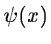and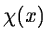such that:(14.69)

is defined. Wg(a,b) denotes the wavelet transform of g with the wavelet function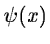: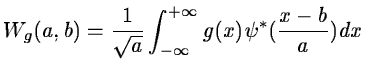(14.70)

We restore g(x) with the wavelet function:(14.71)

The convolution product can be written as: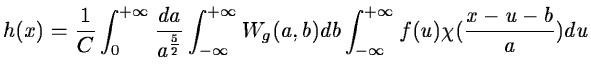(14.72)

Let us denote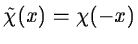. The wavelet transform Wf(a,b) of f(x) with the waveletis: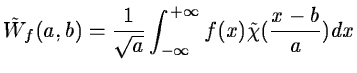(14.73)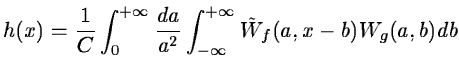(14.74)

Then we get the final result: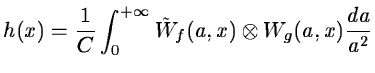(14.75)

In order to compute a convolution with the continuous wavelet transform:
• We compute the wavelet transformof the function f(x) with the wavelet function;
• We compute the wavelet transform Wg(a,b) of the function g(x) with the wavelet function;
• We sum the convolution product of the wavelet transforms, scale by scale.

The wavelet transform permits us to perform any linear filtering. Its efficiency depends on the number of terms in the wavelet transform associated with g(x) for a given signal f(x). If we have a filter where the number of significant coefficients is small for each scale, the complexity of the algorithm is proportional to N. For a classical convolution, the complexity is also proportional to N, but the number of operations is also proportional to the length of the convolution mask. The main advantage of the present technique lies in the possibility of having a filter with long scale terms without computing the convolution on a large window. If we achieve the convolution with the FFT algorithm, the complexity is of order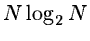. The computing time is longer than the one obtained with the wavelet transform if we concentrate the energy on very few coefficients.Next: The Wiener-like filtering in Up: Noise reduction from the Previous: Noise reduction from the
Petra Nass
1999-06-15